# Image Captioning Using Attention

This example shows how to train a deep learning model for image captioning using attention.

Most pretrained deep learning networks are configured for single-label classification. For example, given an image of a typical office desk, the network might predict the single class "keyboard" or "mouse". In contrast, an image captioning model combines convolutional and recurrent operations to produce a textual description of what is in the image, rather than a single label.

This model trained in this example uses an encoder-decoder architecture. The encoder is a pretrained Inception-v3 network used as a feature extractor. The decoder is a recurrent neural network (RNN) that takes the extracted features as input and generates a caption. The decoder incorporates an attention mechanism that allows the decoder to focus on parts of the encoded input while generating the caption.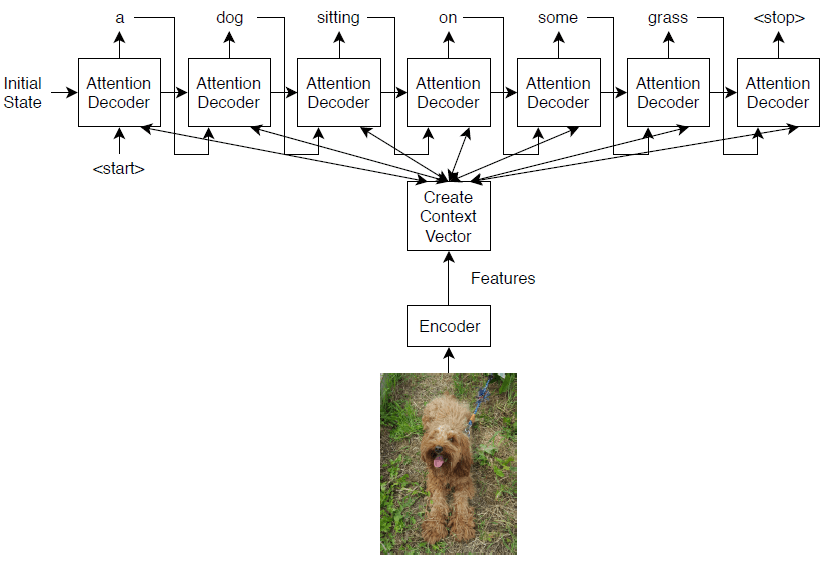The encoder model is a pretrained Inception-v3 model that extracts features from the `"mixed10"` layer, followed by fully connected and ReLU operations.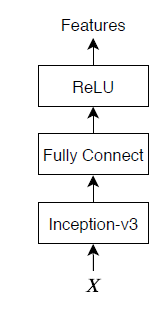The decoder model consists of a word embedding, an attention mechanism, a gated recurrent unit (GRU), and two fully connected operations.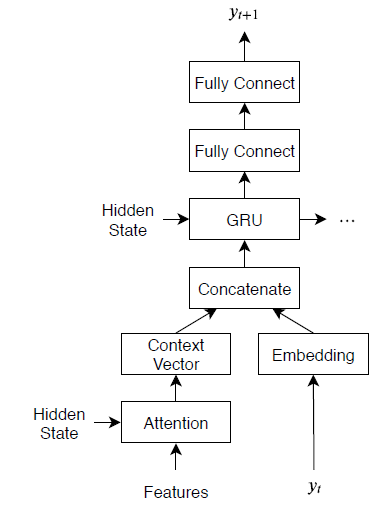Load a pretrained Inception-v3 network. This step requires the Deep Learning Toolbox™ Model for Inception-v3 Network support package. If you do not have the required support package installed, then the software provides a download link.

```net = inceptionv3; inputSizeNet = net.Layers(1).InputSize;```

Convert the network to a `dlnetwork` object for feature extraction and remove the last four layers, leaving the `"mixed10"` layer as the last layer.

```lgraph = layerGraph(net); lgraph = removeLayers(lgraph,["avg_pool" "predictions" "predictions_softmax" "ClassificationLayer_predictions"]);```

View the input layer of the network. The Inception-v3 network uses symmetric-rescale normalization with a minimum value of 0 and a maximum value of 255.

`lgraph.Layers(1)`
```ans = ImageInputLayer with properties: Name: 'input_1' InputSize: [299 299 3] Hyperparameters DataAugmentation: 'none' Normalization: 'rescale-symmetric' NormalizationDimension: 'auto' Max: 255 Min: 0 ```

Custom training does not support this normalization, so you must disable normalization in the network and perform the normalization in the custom training loop instead. Save the minimum and maximum values as doubles in variables named `inputMin` and `inputMax`, respectively, and replace the input layer with an image input layer without normalization.

```inputMin = double(lgraph.Layers(1).Min); inputMax = double(lgraph.Layers(1).Max); layer = imageInputLayer(inputSizeNet,Normalization="none",Name="input"); lgraph = replaceLayer(lgraph,"input_1",layer);```

Determine the output size of the network. Use the `analyzeNetwork` function to see the activation sizes of the last layer. To analyze the network for custom training loop workflows, set the `TargetUsage` option to `"dlnetwork"`.

`analyzeNetwork(lgraph,TargetUsage="dlnetwork")`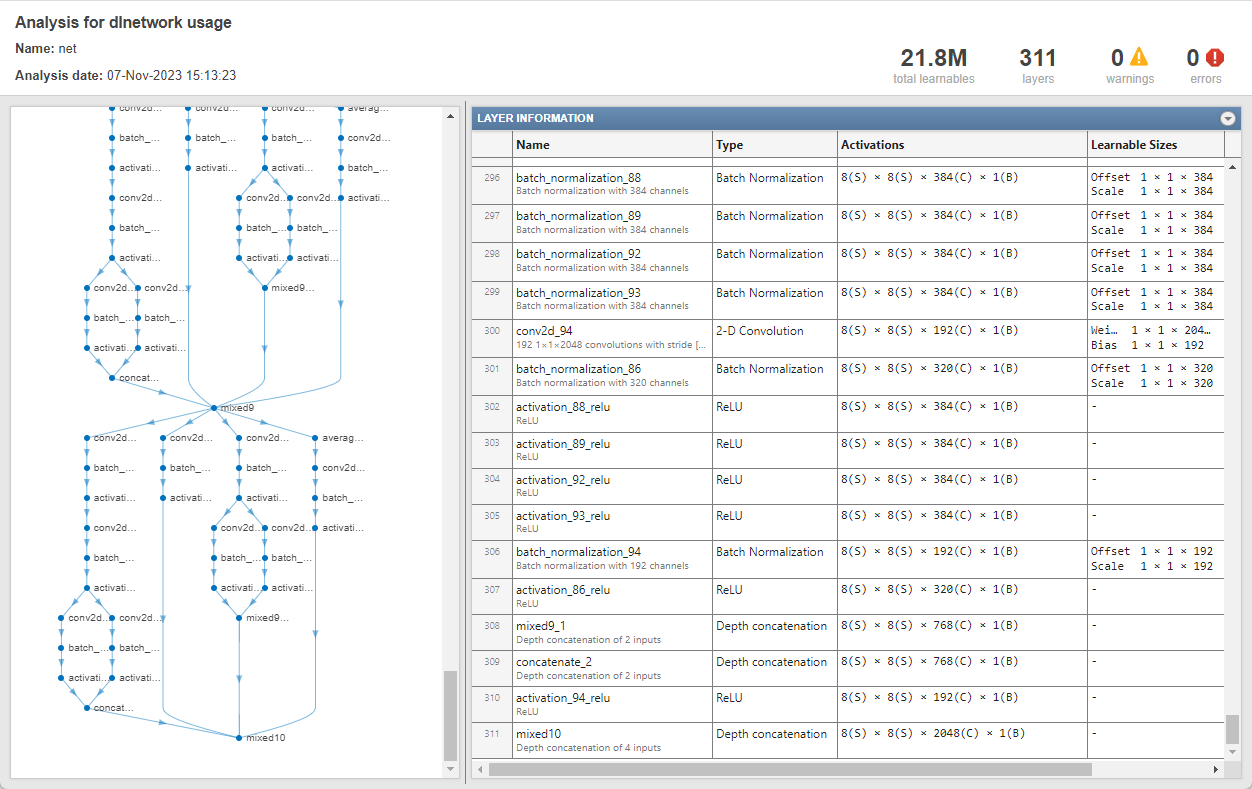Create a variable named `outputSizeNet` containing the network output size.

`outputSizeNet = [8 8 2048];`

Convert the layer graph to a `dlnetwork` object and view the output layer. The output layer is the `"mixed10"` layer of the Inception-v3 network.

`net = dlnetwork(lgraph)`
```dlnet = dlnetwork with properties: Layers: [311×1 nnet.cnn.layer.Layer] Connections: [345×2 table] Learnables: [376×3 table] State: [188×3 table] InputNames: {'input'} OutputNames: {'mixed10'} ```

### Import COCO Data Set

Download images and annotations from the data sets "2014 Train images" and "2014 Train/val annotations," respectively, from https://cocodataset.org/#download. Extract the images and annotations into a folder named `"coco"`. The COCO 2014 data set was collected by Coco Consortium.

Extract the captions from the file `"captions_train2014.json"` using the `jsondecode` function.

```dataFolder = fullfile(tempdir,"coco"); filename = fullfile(dataFolder,"annotations_trainval2014","annotations","captions_train2014.json"); str = fileread(filename); data = jsondecode(str)```
```data = struct with fields: info: [1×1 struct] images: [82783×1 struct] licenses: [8×1 struct] annotations: [414113×1 struct] ```

The `annotations` field of the struct contains the data required for image captioning.

`data.annotations`
```ans=414113×1 struct array with fields: image_id id caption ```

The data set contains multiple captions for each image. To ensure the same images do not appear in both training and validation sets, identify the unique images in the data set using the `unique` function by using the IDs in the `image_id` field of the annotations field of the data, then view the number of unique images.

`numObservationsAll = numel(data.annotations)`
```numObservationsAll = 414113 ```
```imageIDs = [data.annotations.image_id]; imageIDsUnique = unique(imageIDs); numUniqueImages = numel(imageIDsUnique)```
```numUniqueImages = 82783 ```

Each image has at least five captions. Create a struct `annotationsAll` with these fields:

• `ImageID` ⁠— Image ID

• `Filename` ⁠— File name of the image

• `Captions` ⁠— String array of raw captions

• `CaptionIDs` ⁠— Vector of indices of the corresponding captions in `data.annotations`

To make merging easier, sort the annotations by the image IDs.

```[~,idx] = sort([data.annotations.image_id]); data.annotations = data.annotations(idx);```

Loop over the annotations and merge multiple annotations when necessary.

```i = 0; j = 0; imageIDPrev = 0; while i < numel(data.annotations) i = i + 1; imageID = data.annotations(i).image_id; caption = string(data.annotations(i).caption); if imageID ~= imageIDPrev % Create new entry j = j + 1; annotationsAll(j).ImageID = imageID; annotationsAll(j).Filename = fullfile(dataFolder,"train2014","COCO_train2014_" + pad(string(imageID),12,"left","0") + ".jpg"); annotationsAll(j).Captions = caption; annotationsAll(j).CaptionIDs = i; else % Append captions annotationsAll(j).Captions = [annotationsAll(j).Captions; caption]; annotationsAll(j).CaptionIDs = [annotationsAll(j).CaptionIDs; i]; end imageIDPrev = imageID; end```

Partition the data into training and validation sets. Hold out 5% of the observations for testing.

```cvp = cvpartition(numel(annotationsAll),HoldOut=0.05); idxTrain = training(cvp); idxTest = test(cvp); annotationsTrain = annotationsAll(idxTrain); annotationsTest = annotationsAll(idxTest);```

The struct contains three fields:

• `id `— Unique identifier for the caption

• `caption` — Image caption, specified as a character vector

• `image_id` — Unique identifier of the image corresponding to the caption

To view the image and the corresponding caption, locate the image file with file name `"train2014\COCO_train2014_XXXXXXXXXXXX.jpg"`, where `"XXXXXXXXXXXX"` corresponds to the image ID left-padded with zeros to have length 12.

```imageID = annotationsTrain(1).ImageID; captions = annotationsTrain(1).Captions; filename = annotationsTrain(1).Filename;```

To view the image, use the `imread` and `imshow` functions.

```img = imread(filename); figure imshow(img) title(captions) ```

### Prepare Data for Training

Prepare the captions for training and testing. Extract the text from the `Captions` field of the struct containing both the training and test data (`annotationsAll`), erase the punctuation, and convert the text to lowercase.

```captionsAll = cat(1,annotationsAll.Captions); captionsAll = erasePunctuation(captionsAll); captionsAll = lower(captionsAll);```

In order to generate captions, the RNN decoder requires special start and stop tokens to indicate when to start and stop generating text, respectively. Add the custom tokens `"<start>"` and `"<stop>"` to the beginnings and ends of the captions, respectively.

`captionsAll = "<start>" + captionsAll + "<stop>";`

Tokenize the captions using the `tokenizedDocument` function and specify the start and stop tokens using the `CustomTokens` option.

`documentsAll = tokenizedDocument(captionsAll,CustomTokens=["<start>" "<stop>"]);`

Create a `wordEncoding` object that maps words to numeric indices and back. Reduce the memory requirements by specifying a vocabulary size of 5000 corresponding to the most frequently observed words in the training data. To avoid bias, use only the documents corresponding to the training set.

`enc = wordEncoding(documentsAll(idxTrain),MaxNumWords=5000,Order="frequency");`

Create an augmented image datastore containing the images corresponding to the captions. Set the output size to match the input size of the convolutional network. To keep the images synchronized with the captions, specify a table of file names for the datastore by reconstructing the file names using the image ID. To return grayscale images as 3-channel RGB images, set the `ColorPreprocessing` option to `"gray2rgb"`.

```tblFilenames = table(cat(1,annotationsTrain.Filename)); augimdsTrain = augmentedImageDatastore(inputSizeNet,tblFilenames,ColorPreprocessing="gray2rgb")```
```augimdsTrain = augmentedImageDatastore with properties: NumObservations: 78644 MiniBatchSize: 1 DataAugmentation: 'none' ColorPreprocessing: 'gray2rgb' OutputSize: [299 299] OutputSizeMode: 'resize' DispatchInBackground: 0 ```

### Initialize Model Parameters

Initialize the model parameters. Specify 512 hidden units with a word embedding dimension of 256.

```embeddingDimension = 256; numHiddenUnits = 512;```

Initialize a struct containing the parameters for the encoder model.

• Initialize the weights of the fully connected operations using the Glorot initializer, specified by the `initializeGlorot` function, listed at the end of the example. Specify the output size to match the embedding dimension of the decoder (256) and an input size to match the number of output channels of the pretrained network. The `'mixed10'` layer of the Inception-v3 network outputs data with 2048 channels.

```numFeatures = outputSizeNet(1) * outputSizeNet(2); inputSizeEncoder = outputSizeNet(3); parametersEncoder = struct; % Fully connect parametersEncoder.fc.Weights = dlarray(initializeGlorot(embeddingDimension,inputSizeEncoder)); parametersEncoder.fc.Bias = dlarray(zeros([embeddingDimension 1],"single"));```

Initialize a struct containing parameters for the decoder model.

• Initialize the word embedding weights with the size given by the embedding dimension and the vocabulary size plus one, where the extra entry corresponds to the padding value.

• Initialize the weights and biases for the Bahdanau attention mechanism with sizes corresponding to the number of hidden units of the GRU operation.

• Initialize the weights and bias of the GRU operation.

• Initialize the weights and biases of two fully connected operations.

For the model decoder parameters, initialize each of the weighs and biases with the Glorot initializer and zeros, respectively.

```inputSizeDecoder = enc.NumWords + 1; parametersDecoder = struct; % Word embedding parametersDecoder.emb.Weights = dlarray(initializeGlorot(embeddingDimension,inputSizeDecoder)); % Attention parametersDecoder.attention.Weights1 = dlarray(initializeGlorot(numHiddenUnits,embeddingDimension)); parametersDecoder.attention.Bias1 = dlarray(zeros([numHiddenUnits 1],"single")); parametersDecoder.attention.Weights2 = dlarray(initializeGlorot(numHiddenUnits,numHiddenUnits)); parametersDecoder.attention.Bias2 = dlarray(zeros([numHiddenUnits 1],"single")); parametersDecoder.attention.WeightsV = dlarray(initializeGlorot(1,numHiddenUnits)); parametersDecoder.attention.BiasV = dlarray(zeros(1,1,"single")); % GRU parametersDecoder.gru.InputWeights = dlarray(initializeGlorot(3*numHiddenUnits,2*embeddingDimension)); parametersDecoder.gru.RecurrentWeights = dlarray(initializeGlorot(3*numHiddenUnits,numHiddenUnits)); parametersDecoder.gru.Bias = dlarray(zeros(3*numHiddenUnits,1,"single")); % Fully connect parametersDecoder.fc1.Weights = dlarray(initializeGlorot(numHiddenUnits,numHiddenUnits)); parametersDecoder.fc1.Bias = dlarray(zeros([numHiddenUnits 1],"single")); % Fully connect parametersDecoder.fc2.Weights = dlarray(initializeGlorot(enc.NumWords+1,numHiddenUnits)); parametersDecoder.fc2.Bias = dlarray(zeros([enc.NumWords+1 1],"single"));```

### Define Model Functions

Create the functions `modelEncoder` and `modelDecoder`, listed at the end of the example, which compute the outputs of the encoder and decoder models, respectively.

The `modelEncoder` function, listed in the Encoder Model Function section of the example, takes as input an array of activations `X` from the output of the pretrained network and passes it through a fully connected operation and a ReLU operation. Because the pretrained network does not need to be traced for automatic differentiation, extracting the features outside the encoder model function is more computationally efficient.

The `modelDecoder` function, listed in the Decoder Model Function section of the example, takes as input a single input time-step corresponding to an input word, the decoder model parameters, the features from the encoder, and the network state, and returns the predictions for the next time step, the updated network state, and the attention weights.

### Specify Training Options

Specify the options for training. Train for 30 epochs with a mini-batch size of 128 and display the training progress in a plot.

```miniBatchSize = 128; numEpochs = 30; plots = "training-progress";```

Train on a GPU if one is available. Using a GPU requires Parallel Computing Toolbox™ and a supported GPU device. For information on supported devices, see GPU Computing Requirements (Parallel Computing Toolbox).

`executionEnvironment = "auto";`

### Train Network

Train the network using a custom training loop.

At the beginning of each epoch, shuffle the input data. To keep the images in the augmented image datastore and the captions synchronized, create an array of shuffled indices that indexes into both data sets.

For each mini-batch:

• Rescale the images to the size that the pretrained network expects.

• For each image, select a random caption.

• Convert the captions to sequences of word indices. Specify right-padding of the sequences with the padding value corresponding to the index of the padding token.

• Convert the data to `dlarray` objects. For the images, specify dimension labels `"SSCB"` (spatial, spatial, channel, batch).

• For GPU training, convert the data to `gpuArray` objects.

• Extract the image features using the pretrained network and reshape them to the size the encoder expects.

• Evaluate the model loss and gradients using the `dlfeval` and `modelLoss` functions.

• Update the encoder and decoder model parameters using the `adamupdate` function.

• Display the training progress in a plot.

Initialize the parameters for the Adam optimizer.

```trailingAvgEncoder = []; trailingAvgSqEncoder = []; trailingAvgDecoder = []; trailingAvgSqDecoder = [];```

Initialize the training progress plot. Create an animated line that plots the loss against the corresponding iteration.

```if plots == "training-progress" figure lineLossTrain = animatedline(Color=[0.85 0.325 0.098]); xlabel("Iteration") ylabel("Loss") ylim([0 inf]) grid on end```

Train the model.

```iteration = 0; numObservationsTrain = numel(annotationsTrain); numIterationsPerEpoch = floor(numObservationsTrain / miniBatchSize); start = tic; % Loop over epochs. for epoch = 1:numEpochs % Shuffle data. idxShuffle = randperm(numObservationsTrain); % Loop over mini-batches. for i = 1:numIterationsPerEpoch iteration = iteration + 1; % Determine mini-batch indices. idx = (i-1)*miniBatchSize+1:i*miniBatchSize; idxMiniBatch = idxShuffle(idx); % Read mini-batch of data. tbl = readByIndex(augimdsTrain,idxMiniBatch); X = cat(4,tbl.input{:}); annotations = annotationsTrain(idxMiniBatch); % For each image, select random caption. idx = cellfun(@(captionIDs) randsample(captionIDs,1),{annotations.CaptionIDs}); documents = documentsAll(idx); % Create batch of data. [X, T] = createBatch(X,documents,net,inputMin,inputMax,enc,executionEnvironment); % Evaluate the model loss and gradients using dlfeval and the % modelLoss function. [loss, gradientsEncoder, gradientsDecoder] = dlfeval(@modelLoss, parametersEncoder, ... parametersDecoder, X, T); % Update encoder using adamupdate. [parametersEncoder, trailingAvgEncoder, trailingAvgSqEncoder] = adamupdate(parametersEncoder, ... gradientsEncoder, trailingAvgEncoder, trailingAvgSqEncoder, iteration); % Update decoder using adamupdate. [parametersDecoder, trailingAvgDecoder, trailingAvgSqDecoder] = adamupdate(parametersDecoder, ... gradientsDecoder, trailingAvgDecoder, trailingAvgSqDecoder, iteration); % Display the training progress. if plots == "training-progress" D = duration(0,0,toc(start),Format="hh:mm:ss"); addpoints(lineLossTrain,iteration,double(loss)) title("Epoch: " + epoch + ", Elapsed: " + string(D)) drawnow end end end```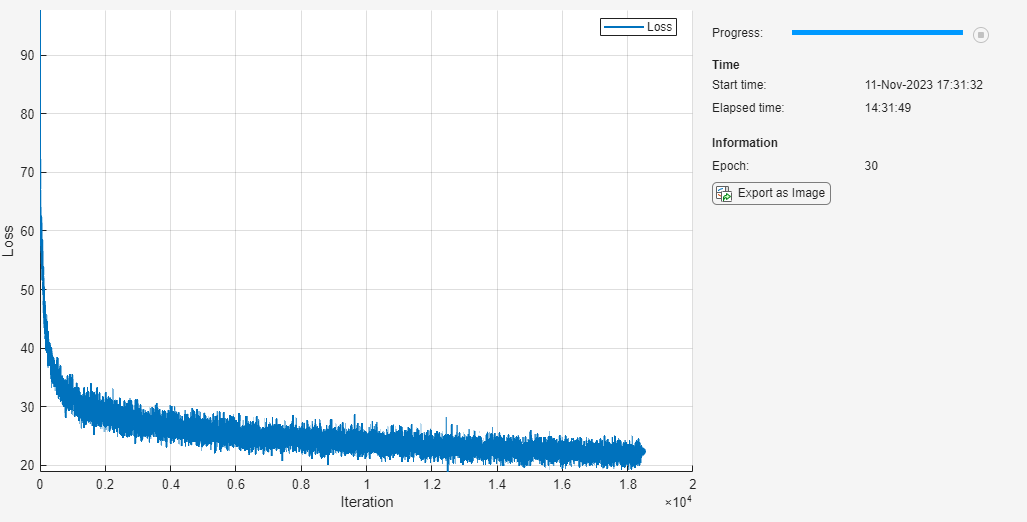### Predict New Captions

The caption generation process is different from the process for training. During training, at each time step, the decoder uses the true value of the previous time step as input. This is known as "teacher forcing". When making predictions on new data, the decoder uses the previous predicted values instead of the true values.

Predicting the most likely word for each step in the sequence can lead to suboptimal results. For example, if the decoder predicts the first word of a caption is "a" when given an image of an elephant, then the probability of predicting "elephant" for the next word becomes much more unlikely because of the extremely low probability of the phrase "a elephant" appearing in English text.

To address this issue, you can use the beam search algorithm: instead of taking the most likely prediction for each step in the sequence, take the top k predictions (the beam index) and for each following step, keep the top k predicted sequences so far according to the overall score.

Generate a caption of a new image by extracting the image features, inputting them into the encoder, and then using the `beamSearch` function, listed in the Beam Search Function section of the example.

```img = imread("laika_sitting.jpg"); X = extractImageFeatures(net,img,inputMin,inputMax,executionEnvironment); beamIndex = 3; maxNumWords = 20; [words,attentionScores] = beamSearch(X,beamIndex,parametersEncoder,parametersDecoder,enc,maxNumWords); caption = join(words)```
```caption = "a dog is standing on a tile floor" ```

Display the image with the caption.

```figure imshow(img) title(caption)```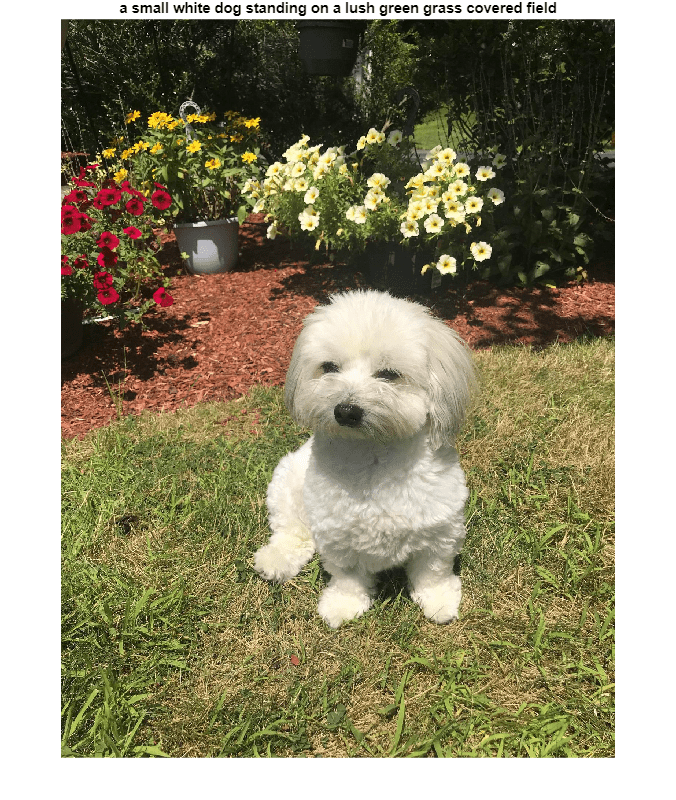### Predict Captions for Data Set

To predict captions for a collection of images, loop over mini-batches of data in the datastore and extract the features from the images using the `extractImageFeatures` function. Then, loop over the images in the mini-batch and generate captions using the `beamSearch` function.

Create an augmented image datastore and set the output size to match the input size of the convolutional network. To output grayscale images as 3-channel RGB images, set the `ColorPreprocessing` option to `"gray2rgb"`.

```tblFilenamesTest = table(cat(1,annotationsTest.Filename)); augimdsTest = augmentedImageDatastore(inputSizeNet,tblFilenamesTest,ColorPreprocessing="gray2rgb")```
```augimdsTest = augmentedImageDatastore with properties: NumObservations: 4139 MiniBatchSize: 1 DataAugmentation: 'none' ColorPreprocessing: 'gray2rgb' OutputSize: [299 299] OutputSizeMode: 'resize' DispatchInBackground: 0 ```

Generate captions for the test data. Predicting captions on a large data set can take some time. If you have Parallel Computing Toolbox™, then you can make predictions in parallel by generating captions inside a `parfor` look. If you do not have Parallel Computing Toolbox. then the `parfor` loop runs in serial.

```beamIndex = 2; maxNumWords = 20; numObservationsTest = numel(annotationsTest); numIterationsTest = ceil(numObservationsTest/miniBatchSize); captionsTestPred = strings(1,numObservationsTest); documentsTestPred = tokenizedDocument(strings(1,numObservationsTest)); for i = 1:numIterationsTest % Mini-batch indices. idxStart = (i-1)*miniBatchSize+1; idxEnd = min(i*miniBatchSize,numObservationsTest); idx = idxStart:idxEnd; sz = numel(idx); % Read images. tbl = readByIndex(augimdsTest,idx); % Extract image features. X = cat(4,tbl.input{:}); X = extractImageFeatures(net,X,inputMin,inputMax,executionEnvironment); % Generate captions. captionsPredMiniBatch = strings(1,sz); documentsPredMiniBatch = tokenizedDocument(strings(1,sz)); parfor j = 1:sz words = beamSearch(X(:,:,j),beamIndex,parametersEncoder,parametersDecoder,enc,maxNumWords); captionsPredMiniBatch(j) = join(words); documentsPredMiniBatch(j) = tokenizedDocument(words,TokenizeMethod="none"); end captionsTestPred(idx) = captionsPredMiniBatch; documentsTestPred(idx) = documentsPredMiniBatch; end```
```Analyzing and transferring files to the workers ...done. ```

To view a test image with the corresponding caption, use the `imshow` function and set the title to the predicted caption.

```idx = 1; tbl = readByIndex(augimdsTest,idx); img = tbl.input{1}; figure imshow(img) title(captionsTestPred(idx)) ```

### Evaluate Model Accuracy

To evaluate the accuracy of the captions using the BLEU score, calculate the BLEU score for each caption (the candidate) against the corresponding captions in the test set (the references) using the `bleuEvaluationScore` function. Using the `bleuEvaluationScore` function, you can compare a single candidate document to multiple reference documents.

The `bleuEvaluationScore` function, by default, scores similarity using n-grams of length one through four. As the captions are short, this behavior can lead to uninformative results as most scores are close to zero. Set the n-gram length to one through two by setting the `NgramWeights` option to a two-element vector with equal weights.

```ngramWeights = [0.5 0.5]; for i = 1:numObservationsTest annotation = annotationsTest(i); captionIDs = annotation.CaptionIDs; candidate = documentsTestPred(i); references = documentsAll(captionIDs); score = bleuEvaluationScore(candidate,references,NgramWeights=ngramWeights); scores(i) = score; end```

View the mean BLEU score.

`scoreMean = mean(scores)`
```scoreMean = 0.4224 ```

Visualize the scores in a histogram.

```figure histogram(scores) xlabel("BLEU Score") ylabel("Frequency")```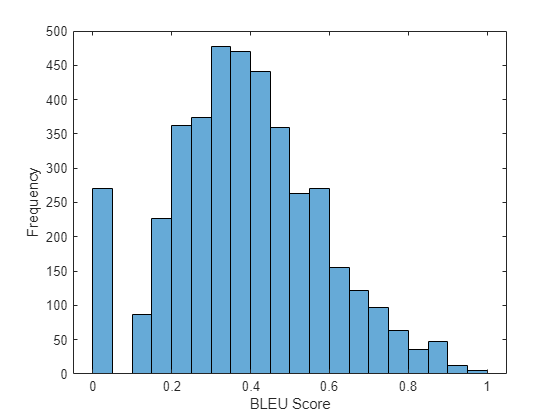### Attention Function

The `attention` function calculates the context vector and the attention weights using Bahdanau attention.

```function [contextVector, attentionWeights] = attention(hidden,features,weights1, ... bias1,weights2,bias2,weightsV,biasV) % Model dimensions. [embeddingDimension,numFeatures,miniBatchSize] = size(features); numHiddenUnits = size(weights1,1); % Fully connect. Y1 = reshape(features,embeddingDimension, numFeatures*miniBatchSize); Y1 = fullyconnect(Y1,weights1,bias1,DataFormat="CB"); Y1 = reshape(Y1,numHiddenUnits,numFeatures,miniBatchSize); % Fully connect. Y2 = fullyconnect(hidden,weights2,bias2,DataFormat="CB"); Y2 = reshape(Y2,numHiddenUnits,1,miniBatchSize); % Addition, tanh. scores = tanh(Y1 + Y2); scores = reshape(scores, numHiddenUnits, numFeatures*miniBatchSize); % Fully connect, softmax. attentionWeights = fullyconnect(scores,weightsV,biasV,DataFormat="CB"); attentionWeights = reshape(attentionWeights,1,numFeatures,miniBatchSize); attentionWeights = softmax(attentionWeights,DataFormat="SCB"); % Context. contextVector = attentionWeights .* features; contextVector = squeeze(sum(contextVector,2)); end```

### Embedding Function

The `embedding` function maps an array of indices to a sequence of embedding vectors.

```function Z = embedding(X, weights) % Reshape inputs into a vector [N, T] = size(X, 1:2); X = reshape(X, N*T, 1); % Index into embedding matrix Z = weights(:, X); % Reshape outputs by separating out batch and sequence dimensions Z = reshape(Z, [], N, T); end```

### Feature Extraction Function

The `extractImageFeatures` function takes as input a trained `dlnetwork` object, an input image, statistics for image rescaling, and the execution environment, and returns a `dlarray` containing the features extracted from the pretrained network.

```function X = extractImageFeatures(net,X,inputMin,inputMax,executionEnvironment) % Resize and rescale. inputSize = net.Layers(1).InputSize(1:2); X = imresize(X,inputSize); X = rescale(X,-1,1,InputMin=inputMin,InputMax=inputMax); % Convert to dlarray. X = dlarray(X,"SSCB"); % Convert to gpuArray. if (executionEnvironment == "auto" && canUseGPU) || executionEnvironment == "gpu" X = gpuArray(X); end % Extract features and reshape. X = predict(net,X); sz = size(X); numFeatures = sz(1) * sz(2); inputSizeEncoder = sz(3); miniBatchSize = sz(4); X = reshape(X,[numFeatures inputSizeEncoder miniBatchSize]); end```

### Batch Creation Function

The `createBatch` function takes as input a mini-batch of data, tokenized captions, a pretrained network, statistics for image rescaling, a word encoding, and the execution environment, and returns a mini-batch of data corresponding to the extracted image features and captions for training.

```function [X, T] = createBatch(X,documents,net,inputMin,inputMax,enc,executionEnvironment) X = extractImageFeatures(net,X,inputMin,inputMax,executionEnvironment); % Convert documents to sequences of word indices. T = doc2sequence(enc,documents,PaddingDirection="right",PaddingValue=enc.NumWords+1); T = cat(1,T{:}); % Convert mini-batch of data to dlarray. T = dlarray(T); % If training on a GPU, then convert data to gpuArray. if (executionEnvironment == "auto" && canUseGPU) || executionEnvironment == "gpu" T = gpuArray(T); end end```

### Encoder Model Function

The `modelEncoder` function takes as input an array of activations `X` and passes it through a fully connected operation and a ReLU operation. For the fully connected operation, operate on the channel dimension only. To apply the fully connected operation across the channel dimension only, flatten the other channels into a single dimension and specify this dimension as the batch dimension using the `DataFormat` option of the `fullyconnect` function.

```function Y = modelEncoder(X,parametersEncoder) [numFeatures,inputSizeEncoder,miniBatchSize] = size(X); % Fully connect weights = parametersEncoder.fc.Weights; bias = parametersEncoder.fc.Bias; embeddingDimension = size(weights,1); X = permute(X,[2 1 3]); X = reshape(X,inputSizeEncoder,numFeatures*miniBatchSize); Y = fullyconnect(X,weights,bias,DataFormat="CB"); Y = reshape(Y,embeddingDimension,numFeatures,miniBatchSize); % ReLU Y = relu(Y); end```

### Decoder Model Function

The `modelDecoder` function takes as input a single time-step `X`, the decoder model parameters, the features from the encoder, and the network state, and returns the predictions for the next time step, the updated network state, and the attention weights.

```function [Y,state,attentionWeights] = modelDecoder(X,parametersDecoder,features,state) hiddenState = state.gru.HiddenState; % Attention weights1 = parametersDecoder.attention.Weights1; bias1 = parametersDecoder.attention.Bias1; weights2 = parametersDecoder.attention.Weights2; bias2 = parametersDecoder.attention.Bias2; weightsV = parametersDecoder.attention.WeightsV; biasV = parametersDecoder.attention.BiasV; [contextVector, attentionWeights] = attention(hiddenState,features,weights1,bias1,weights2,bias2,weightsV,biasV); % Embedding weights = parametersDecoder.emb.Weights; X = embedding(X,weights); % Concatenate Y = cat(1,contextVector,X); % GRU inputWeights = parametersDecoder.gru.InputWeights; recurrentWeights = parametersDecoder.gru.RecurrentWeights; bias = parametersDecoder.gru.Bias; [Y, hiddenState] = gru(Y, hiddenState, inputWeights, recurrentWeights, bias, DataFormat="CBT"); % Update state state.gru.HiddenState = hiddenState; % Fully connect weights = parametersDecoder.fc1.Weights; bias = parametersDecoder.fc1.Bias; Y = fullyconnect(Y,weights,bias,DataFormat="CB"); % Fully connect weights = parametersDecoder.fc2.Weights; bias = parametersDecoder.fc2.Bias; Y = fullyconnect(Y,weights,bias,DataFormat="CB"); end```

### Model Loss

The `modelLoss` function takes as input the encoder and decoder parameters, the encoder features `X`, and the target caption `T`, and returns the loss, the gradients of the encoder and decoder parameters with respect to the loss, and the predictions.

```function [loss,gradientsEncoder,gradientsDecoder,YPred] = ... modelLoss(parametersEncoder,parametersDecoder,X,T) miniBatchSize = size(X,3); sequenceLength = size(T,2) - 1; vocabSize = size(parametersDecoder.emb.Weights,2); % Model encoder features = modelEncoder(X,parametersEncoder); % Initialize state numHiddenUnits = size(parametersDecoder.attention.Weights1,1); state = struct; state.gru.HiddenState = dlarray(zeros([numHiddenUnits miniBatchSize],"single")); YPred = dlarray(zeros([vocabSize miniBatchSize sequenceLength],"like",X)); loss = dlarray(single(0)); padToken = vocabSize; for t = 1:sequenceLength decoderInput = T(:,t); YReal = T(:,t+1); [YPred(:,:,t),state] = modelDecoder(decoderInput,parametersDecoder,features,state); mask = YReal ~= padToken; loss = loss + sparseCrossEntropyAndSoftmax(YPred(:,:,t),YReal,mask); end % Calculate gradients [gradientsEncoder,gradientsDecoder] = dlgradient(loss, parametersEncoder,parametersDecoder); end```

### Sparse Cross Entropy and Softmax Loss Function

The `sparseCrossEntropyAndSoftmax` takes as input the predictions `Y`, corresponding targets `T`, and sequence padding mask, and applies the `softmax` functions and returns the cross-entropy loss.

```function loss = sparseCrossEntropyAndSoftmax(Y, T, mask) miniBatchSize = size(Y, 2); % Softmax. Y = softmax(Y,DataFormat="CB"); % Find rows corresponding to the target words. idx = sub2ind(size(Y), T', 1:miniBatchSize); Y = Y(idx); % Bound away from zero. Y = max(Y, single(1e-8)); % Masked loss. loss = log(Y) .* mask'; loss = -sum(loss,"all") ./ miniBatchSize; end```

### Beam Search Function

The `beamSearch` function takes as input the image features `X`, a beam index, the parameters for the encoder and decoder networks, a word encoding, and a maximum sequence length, and returns the caption words for the image using the beam search algorithm.

```function [words,attentionScores] = beamSearch(X,beamIndex,parametersEncoder,parametersDecoder, ... enc,maxNumWords) % Model dimensions numFeatures = size(X,1); numHiddenUnits = size(parametersDecoder.attention.Weights1,1); % Extract features features = modelEncoder(X,parametersEncoder); % Initialize state state = struct; state.gru.HiddenState = dlarray(zeros([numHiddenUnits 1],"like",X)); % Initialize candidates candidates = struct; candidates.State = state; candidates.Words = "<start>"; candidates.Score = 0; candidates.AttentionScores = dlarray(zeros([numFeatures maxNumWords],"like",X)); candidates.StopFlag = false; t = 0; % Loop over words while t < maxNumWords t = t + 1; candidatesNew = []; % Loop over candidates for i = 1:numel(candidates) % Stop generating when stop token is predicted if candidates(i).StopFlag continue end % Candidate details state = candidates(i).State; words = candidates(i).Words; score = candidates(i).Score; attentionScores = candidates(i).AttentionScores; % Predict next token decoderInput = word2ind(enc,words(end)); [YPred,state,attentionScores(:,t)] = modelDecoder(decoderInput,parametersDecoder,features,state); YPred = softmax(YPred,DataFormat="CB"); [scoresTop,idxTop] = maxk(extractdata(YPred),beamIndex); idxTop = gather(idxTop); % Loop over top predictions for j = 1:beamIndex candidate = struct; candidateWord = ind2word(enc,idxTop(j)); candidateScore = scoresTop(j); if candidateWord == "<stop>" candidate.StopFlag = true; attentionScores(:,t+1:end) = []; else candidate.StopFlag = false; end candidate.State = state; candidate.Words = [words candidateWord]; candidate.Score = score + log(candidateScore); candidate.AttentionScores = attentionScores; candidatesNew = [candidatesNew candidate]; end end % Get top candidates [~,idx] = maxk([candidatesNew.Score],beamIndex); candidates = candidatesNew(idx); % Stop predicting when all candidates have stop token if all([candidates.StopFlag]) break end end % Get top candidate words = candidates(1).Words(2:end-1); attentionScores = candidates(1).AttentionScores; end```

### Glorot Weight Initialization Function

The `initializeGlorot` function generates an array of weights according to Glorot initialization.

```function weights = initializeGlorot(numOut, numIn) varWeights = sqrt( 6 / (numIn + numOut) ); weights = varWeights * (2 * rand([numOut, numIn], "single") - 1); end```